# Units - math word problems

#### Number of problems found: 4326

• Cylinder in cube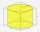Into a paper box in the shape of a cube with an edge of 10 cm is placed a can in the shape of a cylinder with a height of 10 cm and touching all the walls of the cube. What % of the volume of the cube can take up?
• The water tankThe water tank has the shape of a sphere with a radius of 2 m. How many liters of water will fit in the tank? How many kilograms of paint do we need to paint the tank, if we paint with 1 kg of paint 10 m2?
• The corridor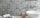The corridor is 12 m long and 3.6 m wide. It must be paved with rectangular tiles measuring 15 cm and 30 cm. Are 1000 pieces of tiles enough to pave the corridor?
• Slow saving in banksHow long will it take to save € 9,000 by depositing € 200 at the beginning of each year at 2% interest?
• Percentage increaseWhat is the annual percentage increase in the city when the population has tripled in 20 years?
• Fruit importsCarriers import 18,324 kg of oranges from Spain. It was 16,857 kg less than mandarins and 2,645 kg more than bananas. a) how many kg did they bring mandarins? b) how many kg did bananas bring? c) how much total fruit?
• Age of familyThe age of father and son is in the ratio 10: 3. The age of the father and daughter is in a ratio of 5: 2. How old are a father and a son if the daughter is 20?
• Magnified cubeIf the lengths of the cube's edges are extended by 5 cm, its volume will increase by 485 cm3. Determine the surface of both the original and the magnified cube.
• AL wireWhat is the weight of an aluminum wire 250 m long with a diameter of 2 mm, if the density of aluminum is p = 2700 kg/m cubic. Determine to the nearest gram.
• Trapezoidal prism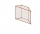Calculate the volume of a prism with a trapezoidal base with side a = 6dm, side c = 4 dm, height of the prism = 8dm. The height of the trapezoid is va = 3dm.
• Brass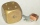Brass is an alloy of copper and zinc in a ratio of 3: 2. How many grams does a component that required 270 g of copper weigh?
• Runcated pyramid teapot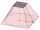The 35 cm high teapot has the shape of a truncated pyramid with the length of the edge of the lower square base a=50 cm and with the edges of the rectangular base b: 20 cm and c: 30 cm. How many liters of water will fit in the teapot?
• Central angleWhat is the length of the arc of a circle with a diameter of 46 cm, which belongs to a central angle of 30°?
• DriversDriver Franta and driver Karel are friends. They leave the start-stop both at the same time in the afternoon - at 13.30. When will they meet again if Franta goes around his route in 50 minutes and Karel in 40 minutes?
• Cyclist 12What is the average speed of a cycle traveling at 20 km in 60 minutes in km/h?
• Ditch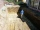The excavator dug 40% of the excavation in 2 hours 20 minutes. How long does it take him to dig the whole ditch?
• Hectoliters of waterThere are 942 hectoliters of water in a cylindrical tank with an inner diameter of 6 m. The water reaches two thirds of the depth of the tank. Calculate its depth.
• Viewing angleThe observer sees a straight fence 60 m long at a viewing angle of 30°. It is 102 m away from one end of the enclosure. How far is the observer from the other end of the enclosure?
• The convoy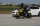During a military exercise, the convoy left the camp at 5 hours at a speed of 40 km/h. Half an hour later, a motorcycle clutch was sent at a speed of 70 km/h. How long before the motorcycle clutch reaches the column?
• Interior angles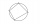In a quadrilateral ABCD, whose vertices lie on some circle, the angle at vertex A is 58 degrees and the angle at vertex B is 134 degrees. Calculate the sizes of the remaining interior angles.

Do you have an exciting math question or word problem that you can't solve? Ask a question or post a math problem, and we can try to solve it.

We will send a solution to your e-mail address. Solved examples are also published here. Please enter the e-mail correctly and check whether you don't have a full mailbox.